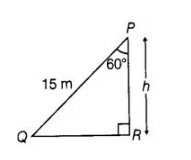# A ladder 15 m long just reaches the top of a vertical wall.

Question:

A ladder 15 m long just reaches the  top of a vertical wall.If the ladders makes an angle of 60° with the wall,then find the height of the wall.

Solution:

Given that,the height of the ladder = 15 m

Let the height of the vertical wall = h

and the ladder makes an angle  of elevation 60° with the wall i.e θ = 60°In $\triangle Q P R$, $\cos 60^{\circ}=\frac{P R}{P Q}=\frac{h}{15}$

$\Rightarrow$ $\frac{1}{2}=\frac{h}{15}$

$\Rightarrow$ $h=\frac{15}{2} \mathrm{~m}$

Hence, the required height of the wall $\frac{15}{2} \mathrm{~m}$.